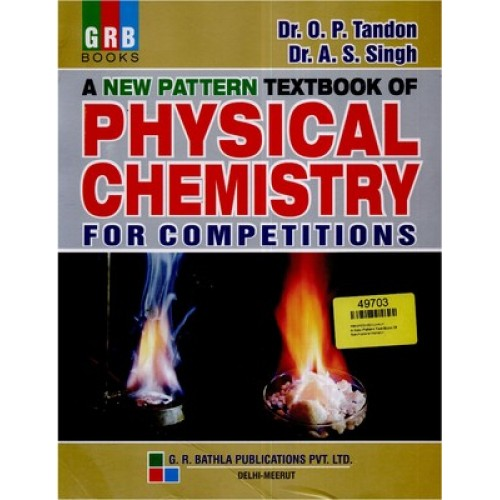You all must have come across a very special book for Physical Chemistry written by Dr. O P Tondon and Dr. A S Singh.

Now here I will be telling you some of the most important questions that came in the previous year IIT JEE and AIPMT exams. This book is having a variety of questions and if sincerely you are going to refer this book for your exam, you will find yourself in an easy and a comfortable zone during the time you will be writing your exam.

Let me first start discussing those important questions.

This question was asked from the chapter Atomic Structure andÃ‚Â Photo chemistry.

Question:Ã‚Â Sunglasses which gets darken when they are exposed to sunlight is found to contain a very small sample Ã‚Â of AgCl(s) which is embedded in the material of glass. When the material is irradiated with light, it will be producing metallic silver and the glass will be darkened. The reaction can be written as:

You can prevent the escape of atoms of chlorine by the rigid glass surface. Also you will find that the reaction gets reverted as and when the source of light is taken away. If tentatively 310 kJ / mol of the energy is needed to forceÃ‚Â Ã‚Â  the reaction to proceed. What should be the wavelength of light that is needed?

Sol. Energy per mole = Energy of one Einstein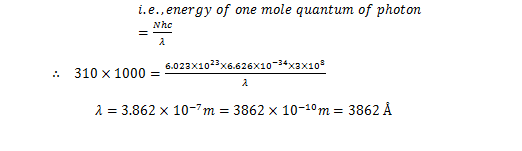Now let us try to come to another important question that has been asked in the exam. This question was taken up from the chapter, Ã¢â‚¬Å“Chemical KineticsÃ¢â‚¬Â and the question can thus be stated as

Ques:

It was found that 10 g atoms of some ÃŽÂ±-active radio isotope were getting disintegrating in a container. In about one hour time, the He gas which could be collected at STP was 11.2 cm3. You now need to calculate the half life of the above mentioned radio isotope.

Sol.

Mass of radioactive isotope = 10 g atoms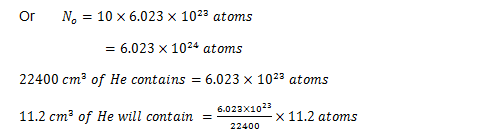Since we can say that we will be getting one atom of helium by the disintegration of just one atom of the given radioisotope, therefore the total countÃ‚Â Ã‚Â Ã‚Â Ã‚Â Ã‚Â  of the atoms of the given radioactive isotope which might have undergone disintegration in one hourMoving in a similar way, we can continue with another important and interesting question from the chapter, Ã¢â‚¬Å“Solutions and the colligative propertiesÃ¢â‚¬Â

Ques:

There was a solution made up of 0.2 g of acetic acid in 20 g of benzene. The freezing point of this solution is found to be lowered byÃ‚Â Ã‚Â Ã‚Â Ã‚Â Ã‚Â Ã‚Â Ã‚Â Ã‚Â Ã‚Â Ã‚Â  0.45Ã‚Â°C. You have been asked to calculate the degree of association of acetic acid in benzene Ã‚Â for benzene is 5.12 kg Ã‚Â Ã‚Â Ã‚Â Ã‚Â  mol-1)

Sol. To do this question, lets first assume the observed molar mass of acetic acid as mobs.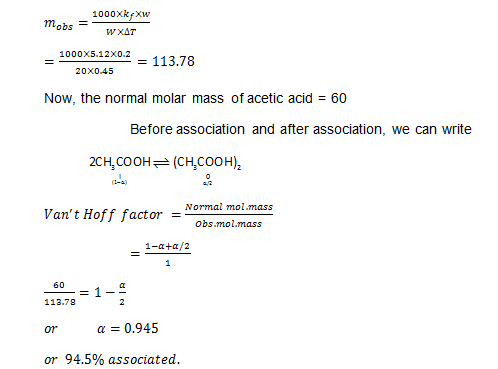So, students as you have seen the above questions have been asked in the previous year JEE papers. You might be thinking what is so special about these questions. These questions are like other questions only but with a clear indication of the importance of the basic Physical Chemistry facts that you need to keep in mind before you start attempting any such questions.

Coming to the other questions in a similar fashion, I found these interesting ones for you.

QuesÃ‚Â When a mixture of 1 Ã¢â‚¬â€œ pentyne (A) was Ã‚Â treated with the solution of 4N alcoholic KOH at 175Ã‚Â°C, it was found to be converting into Ã‚Â Ã‚Â Ã‚Â Ã‚Â Ã‚Â Ã‚Â Ã‚Â Ã‚Â Ã‚Â Ã‚Â  an equilibrium mixture of 1.3% of 1- pentyne (A), 95.2%Ã‚Â  2 Ã¢â‚¬â€œ pentyne (B) and 3.5% of 1,2 Ã¢â‚¬â€œ Ã‚Â  Ã‚Â  Ã‚Â pentadiene (C). The mixture at the equilibrium was then maintained at the given temperature of 175Ã‚Â°C. Ã‚Â As a matter of fact, you now need to find out the Ã¢Ë†â€ GÃ‚Â° for the following reactions: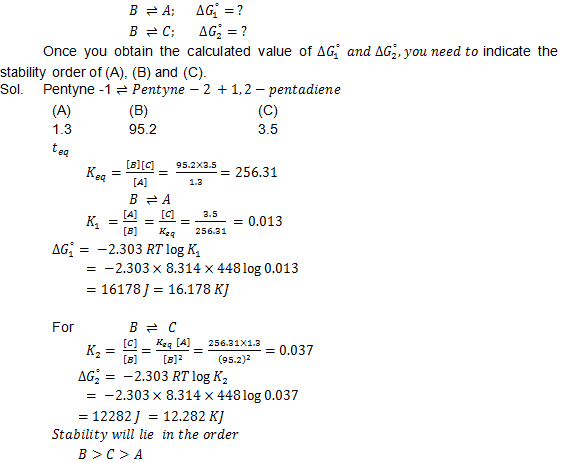Ques.Ã‚Â  It was found that on the introduction of Ã‚Â 3.06 g of solid NH4 HS into a two litre flask at 27Ã‚Â°C, 30% of the given solid got decomposed into ammonia and hydrogen sulphide

(i) First you have to find out Ã‚Â KC and KP for the Ã‚Â  reaction at the given temperature

(ii) Secondly you need to justify what is going to happen to the equilibrium condition when moreÃ‚Â  NH4 HS will be added into the flask?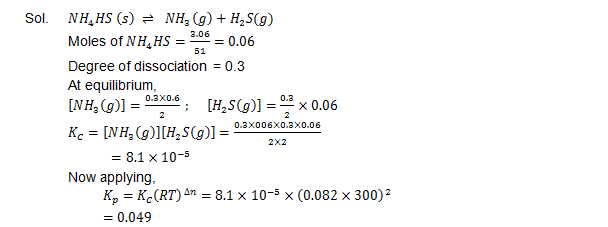As per the known facts, NH4HS is solid, so it is not going cause any change in equilibrium.Students, so far I have tried to discuss these five important questions from the previous year exams.Ã‚Â Now I think, you would have got the idea of what type of questions are generally asked in the exam.Ã‚Â With this basic idea in mind, you can now proceed with the other half of this article.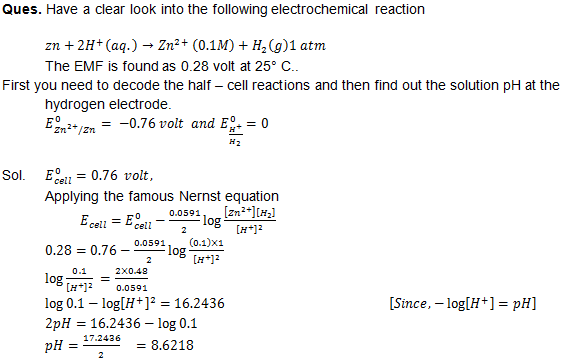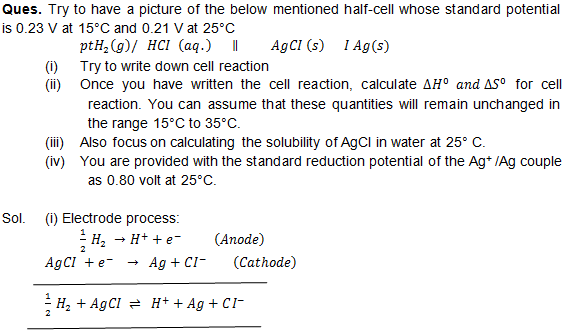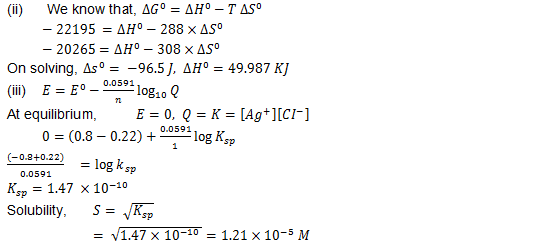Students, now let me come over to the another valuable question.

Ques

There was a hydrogenation reaction which was carried out at 500 K. If hypothetically, the same reaction will be allowed to be carried out in presence Ã‚Â Ã‚Â Ã‚Â Ã‚Â  of a catalyst and that too maintaining the rate to be the same, the temperature that will be required will be approx. 400 k. You now need to find out the activation energy Ã‚Â  of this Ã‚Â reaction if it is taken that the catalyst will lower the activation energy barriers by 20 kJ mol-1.

According to the Ã‚Â Arrhenius equation,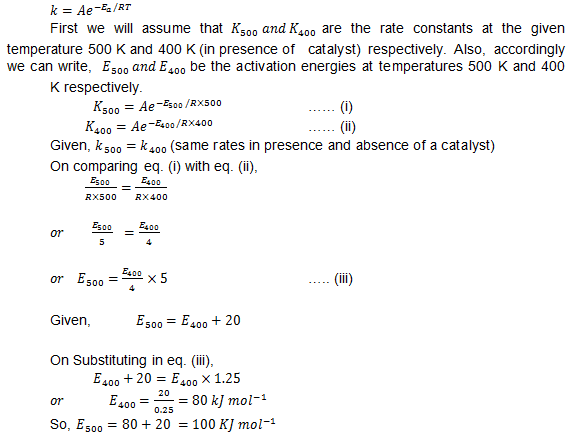Now I think finally we can come over to the last two important questions in queue. These are: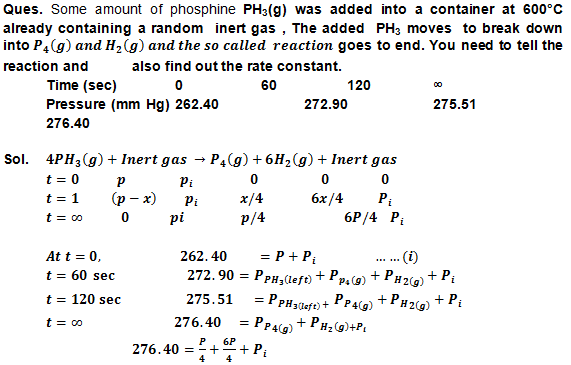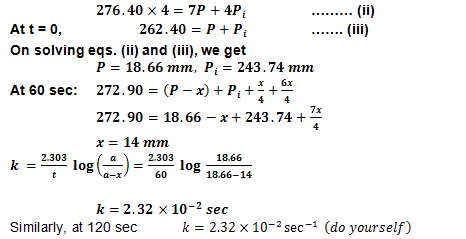Since, Ã¢Ë†Âµ since the values of Ã¢â‚¬ËœkÃ¢â‚¬â„¢ are found to be same, thus the reaction can be said to belong to first order.

Ques. There are a series of changes to which one mole of an ideal gas is put through. These changes have been depicted in the graph in which A, B and C indicate the three different stages of this system. We have shown all the variables at all the stages in the graph.

(a)Ã‚Â  First of all you need to calculate the pressure at these three stages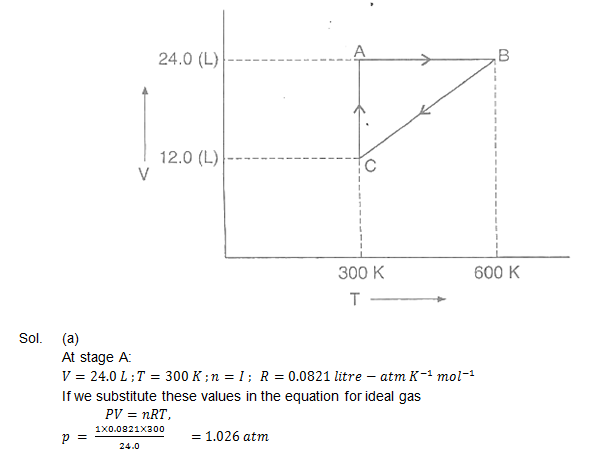At stage B:

Since from the graph it is clear that the volume remains exactly the same but there is a change in the temperature from 300 K to 600 K. Therefore, according to the law of pressure, the pressure will be two times Ã‚Â at B as compared to Ã‚Â A.

Pressure at B =Ã‚Â At stage C:

From the graph we can see that the temperature is 330 K and the corresponding volume is Ã‚Â½Ã‚Â  that of A. Therefore, now again taking the BoyleÃ¢â‚¬â„¢s law, the pressure at C can be said to be two times Ã‚Â with respect to A.So students, these are some of the most important questions that have been asked in the previous year exams. I have tried to tell you the questions in detail so a few of them, although were objective types, were explained in little subjective way.

So, now what you need to do is to go through the concepts and the explanations in details, so that not only these 10 questions, but you should be able to cover almost all the questions of similar types.

Now I will be taking a leave to meet you again with another interesting chemistry stuff.

Thanks for rating this! Now tell the world how you feel - .
How does this post make you feel?
• Excited
• Fascinated
• Amused
• Bored